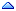Home » SQL & PL/SQL » SQL & PL/SQL » Help with Logic !!!!!!!!
Help with Logic !!!!!!!! Wed, 30 January 2002 23:55Rm69 Messages: 39Registered: January 2002 Member
q) How can l get this proc to sum up the capital for each branch and group it by branch and brockercd or branch and sbrockercd ? l l have a branch field in my table.

q) How can l insert the value passed to parameter v_start_date to a column change_date in the same table?

Procedure Mis_WrkFlow_Cap_Proc
(v_start_date DATE,v_end_date DATE)

AS

BEGIN

rules,returned,disburse,active,pre_reject,rejected,CAPTURE_DATE)

rules.capital,returned.capital,disburse.capital,active.capital,pre_reject.capital,rejected.capital,SYSDATE

from (SELECT sum(CAPITAL) capital
from ZW30800P
where SCDATE between v_start_date and v_end_date) received,

(SELECT sum(CAPITAL) capital
from ZW30800P
where STATUS = 'PRE'
and PQDATE between v_start_date and v_end_date) prequota,

(SELECT sum(CAPITAL) capital
from ZW30800P
where STATUS = 'BAT'
and BADATE between v_start_date and v_end_date) batched,

(SELECT sum(CAPITAL) capital
from ZW30800P
where STATUS = 'SCA'
and SCDATE between v_start_date and v_end_date) scanned_received,

(SELECT sum(CAPITAL) capital
from ZW30800P
where STATUS = 'CAP'
and CAPDATE between v_start_date and v_end_date) captured,

(SELECT sum(CAPITAL) capital
from ZW30800P
where STATUS = 'CHK'
and CHKDATE between v_start_date and v_end_date) checked,

(SELECT sum(CAPITAL) capital
from ZW30800P
where STATUS = 'RUL'
and LASTCHGDAT between v_start_date and v_end_date) rules,

(SELECT sum(CAPITAL) capital
from ZW30800P
where STATUS = 'ERR'
and RETDATE between v_start_date and v_end_date) returned,

(SELECT sum(CAPITAL) capital
from ZW30800P
where STATUS = 'DIS'
and LASTCHGDAT between v_start_date and v_end_date) disburse,

(SELECT sum(CAPITAL) capital
from ZW30800P
where STATUS = 'ACT'
and DISDATE between v_start_date and v_end_date) active,

(SELECT sum(CAPITAL) capital
from ZW30800P
where STATUS = 'REF'
and PRDATE between v_start_date and v_end_date) pre_reject,

(SELECT sum(CAPITAL) capital
from ZW30800P
where STATUS = 'REJ'
and REJDATE between v_start_date and v_end_date) rejected;

end;
Re: Help with Logic !!!!!!!! [message #18455 is a reply to message #18432] Thu, 31 January 2002 08:00sokeh Messages: 77Registered: August 2000 Member
I still don't see how you can make this query work.
insert into table(branch)
select branch.branch from another table
unless is branch that precedes the dot(.) is a table or is of an object type.
In any case, you can use ROLLUP to sum each branch.
There are other ways but I will try rollup first.
 Previous Topic: Good PARTITIONING PRACTICE.. How do i automate partitioning? Next Topic: How can I use count(*) with out sorting ?
Goto Forum:

Current Time: Thu Sep 21 11:25:33 CDT 2023